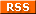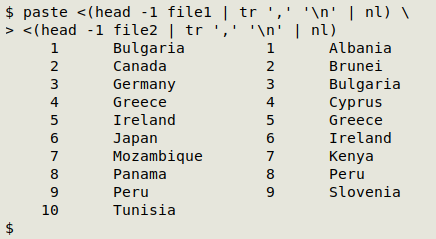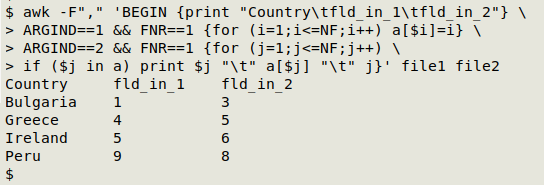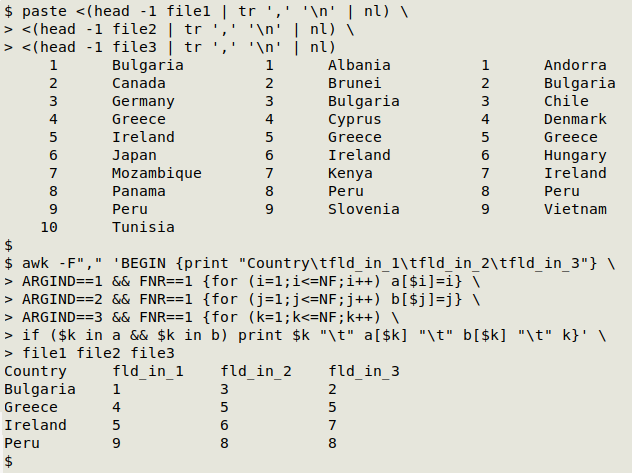For a full list of BASHing data blog posts see the index page.# How to build a multi-file fields concordance

Suppose I have 2 CSV tables with some shared fields, like this:

file1:
nnn,nnn,nnn,nnn,nnn,nnn,nnn,nnn,nnn,nnn
nnn,nnn,nnn,nnn,nnn,nnn,nnn,nnn,nnn,nnn
nnn,nnn,nnn,nnn,nnn,nnn,nnn,nnn,nnn,nnn

file2:
Albania,Brunei,Bulgaria,Cyprus,Greece,Ireland,Kenya,Peru,Slovenia
nnn,nnn,nnn,nnn,nnn,nnn,nnn,nnn,nnn
nnn,nnn,nnn,nnn,nnn,nnn,nnn,nnn,nnn
nnn,nnn,nnn,nnn,nnn,nnn,nnn,nnn,nnn

Suppose also I'd like to compare what's in the shared fields, using a tool like AWK. That means I'll need the field numbers. Those numbers are easy to get with shell tools:

paste <(head -1 file1 | tr ',' '\n' | nl) \
<(head -1 file2 | tr ',' '\n' | nl)But simple lists of fields aren't eyeball-friendly. What I really want is a concordance: a table listing just the shared fields with their respective numbers in the 2 files. This AWK command will build a concordance:

awk -F"," 'BEGIN {print "Country\tfld_in_1\tfld_in_2"} \
ARGIND==1 && FNR==1 {for (i=1;i<=NF;i++) a[\$i]=i} \
ARGIND==2 && FNR==1 {for (j=1;j<=NF;j++) \
if (\$j in a) print \$j "\t" a[\$j] "\t" j}' file1 file2The 2 files are comma-separated, so the AWK command begins by specifying the input field separator: -F",".

In a BEGIN statement, AWK is told to print the tab-separated header line for the concordance: print "Country\tfld_in_1\tfld_in_2".

AWK will work on both "file1" and "file2", because both are arguments for the command. Here I've distinguished the two arguments using AWK's ARGIND variable.

When ARGIND==1, AWK looks at the first file, but the second condition is that only the first line (the header) is processed: ARGIND==1 && FNR==1. The action when these two conditions are met is that AWK loops through each of the fields in "file1" (for (i=1;i<=NF;i++)) and builds an array "a" whose index string is the country name (the field name) and whose value string is the field number: a[\$i]=i.

AWK continues processing "file1" but finds no more to do, since the condition for action only applies to the first line. Next, AWK moves to "file2", and again the condition is that only the first line is to be processed: ARGIND==2 && FNR==1. AWK loops through each of the fields in "file2" (for (j=1;j<=NF;j++)), but this time the action is based on an if condition. If the current field's contents are an index string in array "a" (if (\$j in a)), then AWK prints the current field's contents (a country name), a tab, the field number associated with the field contents in array "a", a tab, and the field number of the current field (print \$j "\t" a[\$j] "\t" j).

If the country name in the "file2" header isn't an index string in array "a", AWK moves on to the next field in the header without doing anything.

I've used the ARGIND method for identifying files because that command structure is easily extended. Suppose there's a third file, "file3", with the same shared fields:

file3:
Andorra,Bulgaria,Chile,Denmark,Greece,Hungary,Ireland,Peru,Vietnam
nnn,nnn,nnn,nnn,nnn,nnn,nnn,nnn,nnn
nnn,nnn,nnn,nnn,nnn,nnn,nnn,nnn,nnn
nnn,nnn,nnn,nnn,nnn,nnn,nnn,nnn,nnn

To make a 3-file concordance, I only need to build a second array "b" from the "file2" header, then extend the "file3" condition: if the current field contents is an index string in "a" and an index string in "b"...

awk -F"," 'BEGIN {print "Country\tfld_in_1\tfld_in_2\tfld_in3"} \
ARGIND==1 && FNR==1 {for (i=1;i<=NF;i++) a[\$i]=i} \
ARGIND==2 && FNR==1 {for (j=1;j<=NF;j++) b[\$j]=j} \
ARGIND==3 && FNR==1 {for (k=1;k<=NF;k++) \
if (\$k in a && \$k in b) print \$k "\t" a[\$k] "\t" b[\$k] "\t" k}' \
file1 file2 file3Last update: 2020-12-23
The blog posts on this website are licensed under a